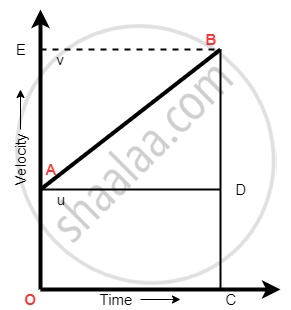Share

# Equations of Motion by Graphical Method - Equation for Velocity-time Relation

#### notes

Equation of velocity – Time Relation (1st equation of motion)
Slope of velocity – Time graph = AB = Acceleration

"Slope" = "Rise" / "Run""AB"= "BD" /"AD"            ...(BC=BD + CD)

"AB" = ("BC" – "CD")/ "AD"  ...(BD = BC – CD)

"a" =("v" – "u") / "t"

at = v – u

at + u = v

v = u + at

S